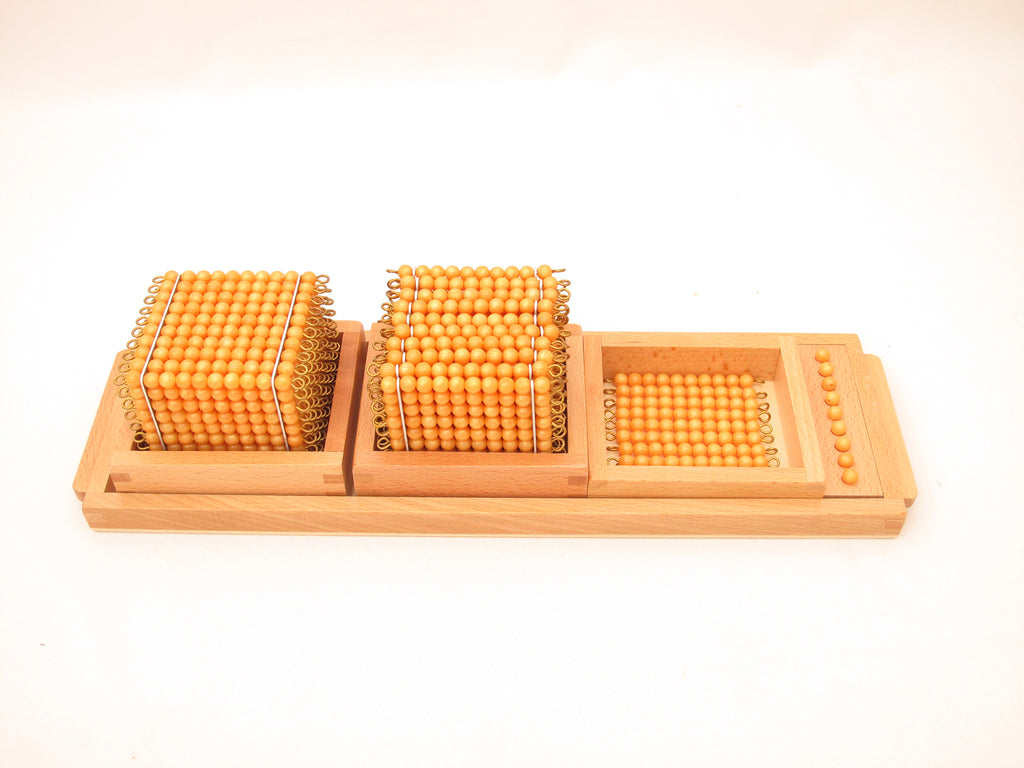### Introduction to Decimal Symbols

\$72.90 USD

Montessori Mathematics Material

The golden bead units, ten bars, hundred squares, and thousand cubes are used mainly in Montessori decimal system to demonstrate values of ones, tens, hundreds, and thousands; to associate beads to numbers; and to operate mathematical equations in addition, subtraction, multiplication and division.

What's Included:

• A wooden tray
• nine unit beads on a holder
• nine bead bar of tens in a tray
• nine hundred squares in a tray
• one thousand cube in a tray

Sale

Unavailable

Sold Out